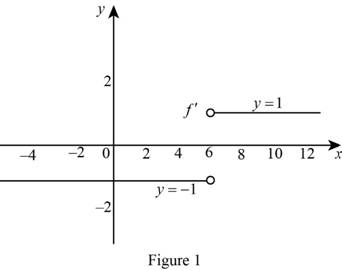# The function f ( x ) = | x − 6 | is not differentiable at x = 6 and the formula of derivative of f ( x ) .### Single Variable Calculus: Concepts...

4th Edition
James Stewart
Publisher: Cengage Learning
ISBN: 9781337687805### Single Variable Calculus: Concepts...

4th Edition
James Stewart
Publisher: Cengage Learning
ISBN: 9781337687805

#### Solutions

Chapter 2.7, Problem 51E
To determine

## To show: The function f(x)=|x−6| is not differentiable at x=6 and the formula of derivative of f(x).

Expert Solution

### Explanation of Solution

Definition used:

An absolute function |x| is defined as follows.

|x|={x if x<0x if x0

Proof:

Rewrite the function f(x) in terms of a piecewise function.

Use the definition of absolute value function to define the function |x6|,

f(x)={(x6)if x6<0x6if x60={x+6if x<6x6if x6

The left hand derivative at x=6 is calculated as follows,

f(6)=limx6f(x)f(6)x6

Since x tends to 6, x<6

f(6)=limx6x+6(6+6)x6=limx6x+6x6=limx6(x6)(x6)=1

Thus, f(6)=1. (1)

The right hand derivative at x=6 is calculated as follows,

f+(6)=limx6+f(x)f(6)x6

Since x tends to 6+,x>6

f+(6)=limx6+x6(66)x6=limx6+x6x6=1

Thus, f+(6)=1. (2)

From the equation (1) and (2), f(6)f+(6).

This implies that, the value of f(6) does not exist.

Therefore, the function f(x)={x+6if x<6x6if x6 is not differentiable at x=6

Hence, the required proof is obtained.

Now find the formula of derivative of f(x).

From equation (1) and (2), f(6)=1 and f+(6)=1.

This implies that, the function f(x)=1 at x<6 and f(x)=1 at x>6.

Thus the required derivative function is, f(x)={1if x<61if x>6.

Graph:

Use the above information to draw the graph of f as shown below in Figure 1.Thus,f is the required graph.

### Have a homework question?

Subscribe to bartleby learn! Ask subject matter experts 30 homework questions each month. Plus, you’ll have access to millions of step-by-step textbook answers!